Скачать презентацию EXAMPLE 6 Find probability of three dependent events

d133efa2a824052bedb4f70d025bf7b4.ppt

• Количество слайдов: 7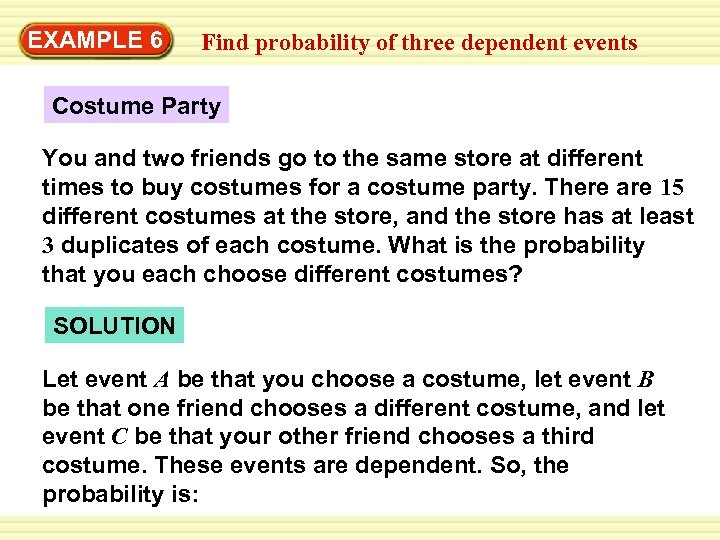EXAMPLE 6 Find probability of three dependent events Costume Party You and two friends go to the same store at different times to buy costumes for a costume party. There are 15 different costumes at the store, and the store has at least 3 duplicates of each costume. What is the probability that you each choose different costumes? SOLUTION Let event A be that you choose a costume, let event B be that one friend chooses a different costume, and let event C be that your other friend chooses a third costume. These events are dependent. So, the probability is: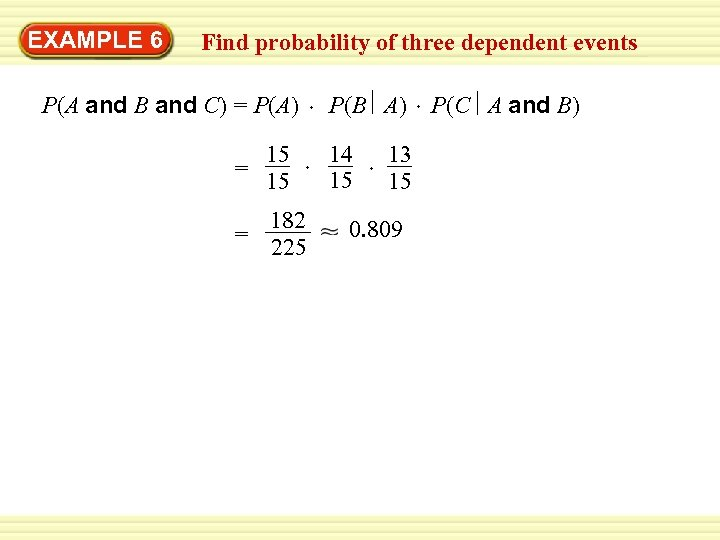EXAMPLE 6 Find probability of three dependent events P(A and B and C) = P(A) = 15 15 = 182 225 P(B A) 14 15 13 15 0. 809 P(C A and B)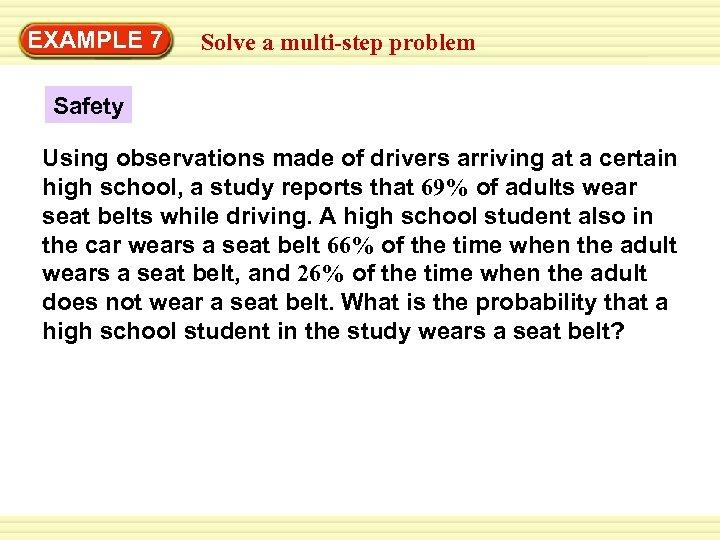EXAMPLE 7 Solve a multi-step problem Safety Using observations made of drivers arriving at a certain high school, a study reports that 69% of adults wear seat belts while driving. A high school student also in the car wears a seat belt 66% of the time when the adult wears a seat belt, and 26% of the time when the adult does not wear a seat belt. What is the probability that a high school student in the study wears a seat belt?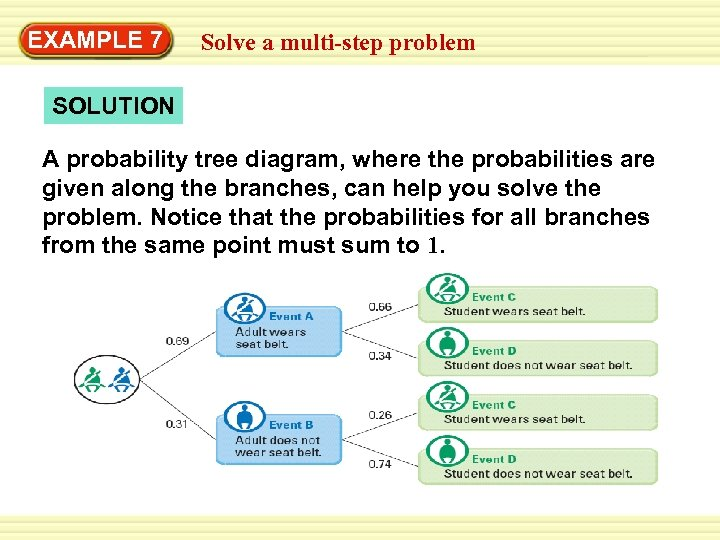EXAMPLE 7 Solve a multi-step problem SOLUTION A probability tree diagram, where the probabilities are given along the branches, can help you solve the problem. Notice that the probabilities for all branches from the same point must sum to 1.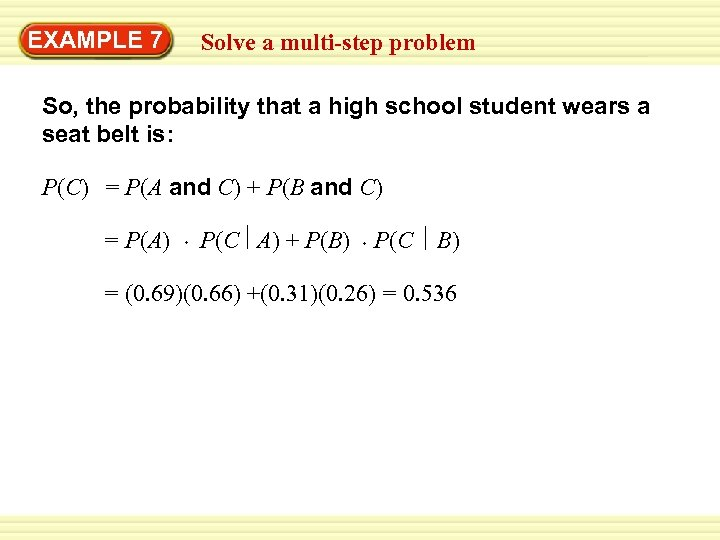EXAMPLE 7 Solve a multi-step problem So, the probability that a high school student wears a seat belt is: P(C) = P(A and C) + P(B and C) = P(A) P(C A) + P(B) P(C B) = (0. 69)(0. 66) +(0. 31)(0. 26) = 0. 536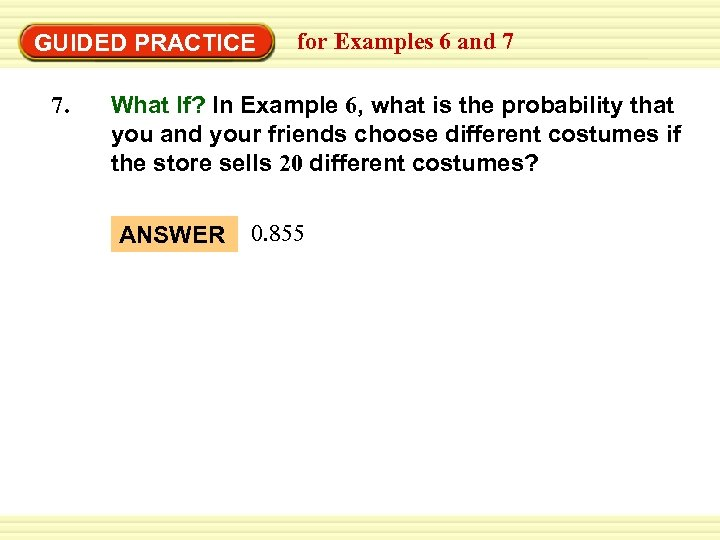GUIDED PRACTICE 7. for Examples 6 and 7 What If? In Example 6, what is the probability that you and your friends choose different costumes if the store sells 20 different costumes? ANSWER 0. 855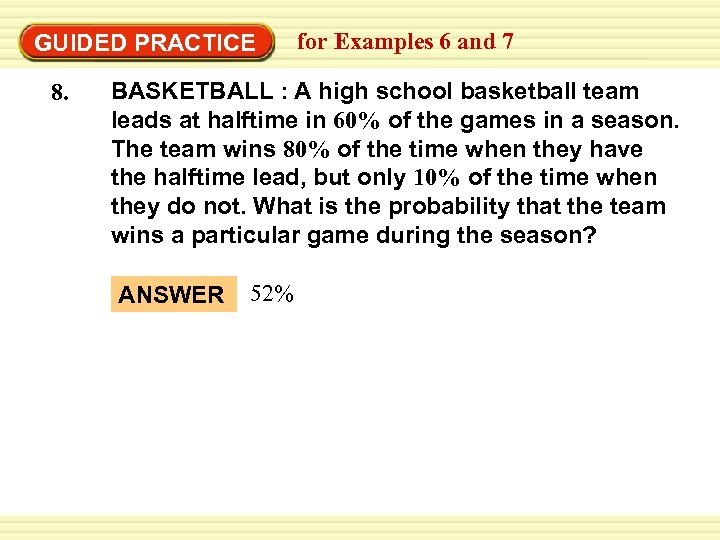GUIDED PRACTICE 8. for Examples 6 and 7 BASKETBALL : A high school basketball team leads at halftime in 60% of the games in a season. The team wins 80% of the time when they have the halftime lead, but only 10% of the time when they do not. What is the probability that the team wins a particular game during the season? ANSWER 52%